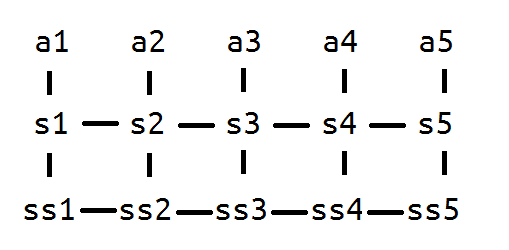# 【计数】cf223C. Partial Sums

You've got an array a, consisting of n integers. The array elements are indexed from 1 to n. Let's determine a two step operation like that:

1. First we build by the array a an array s of partial sums, consisting of n elements. Element number i (1 ≤ i ≤ n) of array s equals. The operation x mod y means that we take the remainder of the division of number x by number y.
2. Then we write the contents of the array s to the array a. Element number i (1 ≤ i ≤ n) of the array s becomes the i-th element of the array a (ai = si).

You task is to find array a after exactly k described operations are applied.

## Input

The first line contains two space-separated integers n and k (1 ≤ n ≤ 2000, 0 ≤ k ≤ 109). The next line contains n space-separated integers a1, a2, ..., an — elements of the array a (0 ≤ ai ≤ 109).

## Output

Print n integers  — elements of the array a after the operations are applied to it. Print the elements in the order of increasing of their indexes in the array a. Separate the printed numbers by spaces.

## 题目分析

3.16upd：1 #include<bits/stdc++.h>
2 #define MO 1000000007
3 const int maxn = 2035;
4
5 int n,k,a[maxn],b[maxn],inv[maxn],fac[maxn],pre[maxn];
6
7 void init()
8 {
9     fac = fac = inv = inv = 1;
10     for (int i=2; i<=n; i++)
11         fac[i] = 1ll*fac[i-1]*i%MO,
12         inv[i] = MO-1ll*(MO/i)*inv[MO%i]%MO;
13     pre = 1;
14     for (int i=1; i<=n; i++)
15         pre[i] = 1ll*pre[i-1]*(k%MO+i-1)%MO*inv[i]%MO;
16 }
17 int main()
18 {
19     scanf("%d%d",&n,&k);
20     for (int i=1; i<=n; i++) scanf("%d",&a[i]), b[i] = a[i];
21     if (k){
22         init();
23         for (int i=1; i<=n; i++)
24         {
25             b[i] = 0;
26             for (int j=1; j<=i; j++)
27                 b[i] = 1ll*(b[i]+1ll*pre[i-j]*a[j]%MO)%MO;
28         }
29     }
30     for (int i=1; i<=n; i++) printf("%d ",b[i]);
31     return 0;
32 }

END

posted @ 2019-03-15 21:25  AntiQuality  阅读(147)  评论(0编辑  收藏